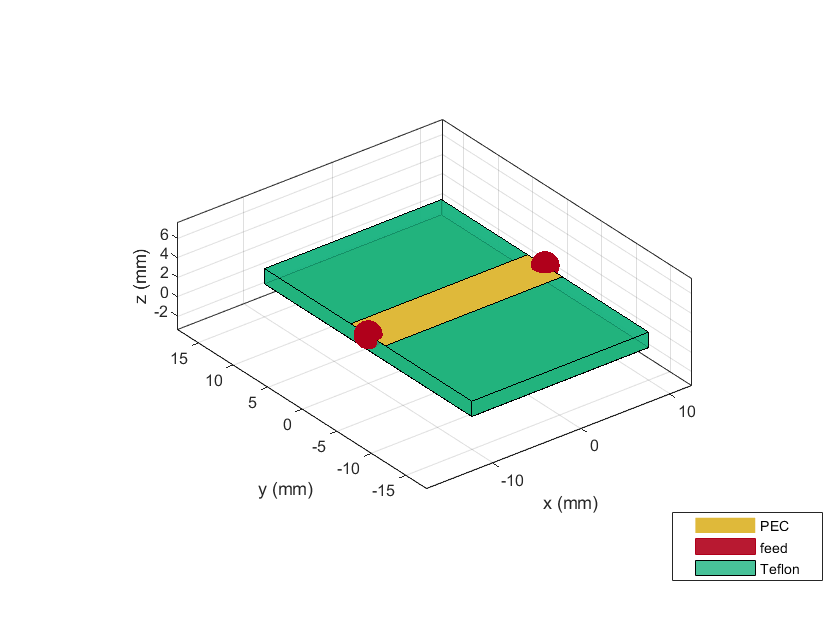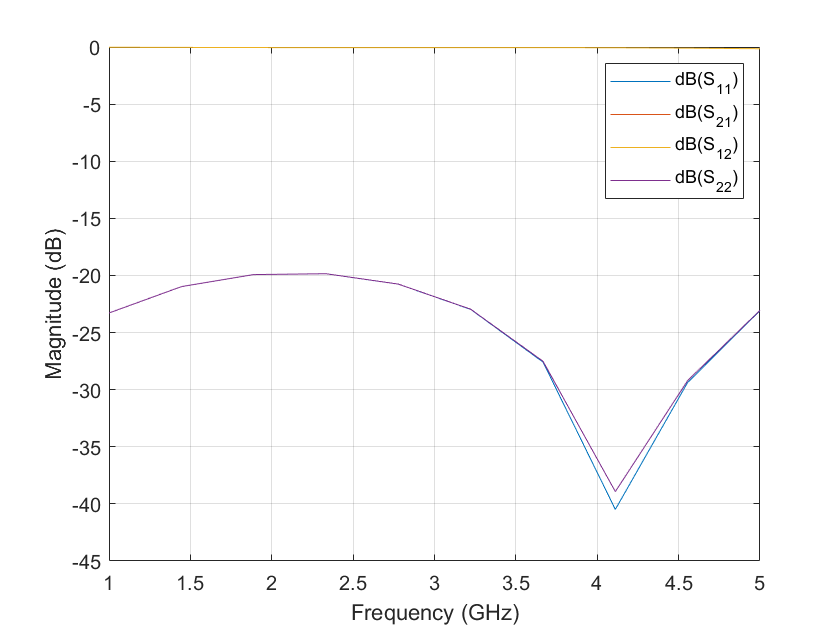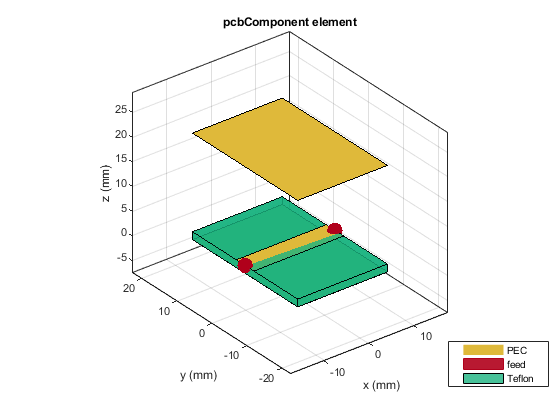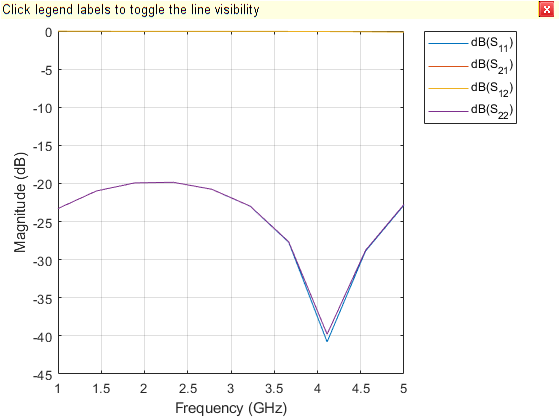# pcbComponent

Create single or multifeed PCB component

## Description

Use the `pcbComponent` object to create a multiport PCB component consisting of metal and dielectric layers.

## Creation

### Syntax

``pcb = pcbComponent``
``pcb = pcbComponent(Name=Value)``

### Description

example

``pcb = pcbComponent` creates a two-port PCB component.`
````pcb = pcbComponent(Name=Value)` sets Properties using one or more name-value arguments. For example, ```pcb = pcbComponent(Name=PCBWilkinson)``` creates a PCB component named `'PCBWilkinson'` Properties not specified retain their default values.```

## Properties

expand all

Name of the PCB component, specified a character vector or string scalar.

Example: ```component = pcbComponent(Name='PCBsplitter')```

Data Types: `char` | `string`

Design revision details of the PCB component, specified as a character vector or string scalar.

Example: `component = pcbComponent(Revision='v2.0')`

Data Types: `char` | `string`

Shape of the PC board, specified as a shape object. You can specify any one of the shapes from Custom Geometry and PCB Fabrication.

Example: ```trace = tracerectangular; component = pcbComponent(BoardShape=trace)``` creates a rectangle shaped trace on a PCB.

Data Types: `char` | `string`

Height of the PCB component, specified as a positive scalar in meters. To understand more about `BoardThickness`, see Board Thickness versus Dielectric Thickness in PCB.

Example: ```component = pcbComponent(BoardThickness=0.0026)```

Data Types: `double`

Metal and dielectric layers, specified a cell array of metal and dielectric layers. You can specify one metal shape or one dielectric substrate per layer starting with the top layer and proceeding downward.

Data Types: `cell`

Feed locations on the PCB component in Cartesian coordinates, specified as either an N-by-3 or N-by-4 array with N representing the number for ports on the PCB component. You can place the feed inside the board or at the edge of the board. The arrays translate into the following:

• N-by-3 – [x, y, Layer]

• N-by-4 – [x, y, SigLayer, GndLayer]

Example: ```component = pcbComponent(FeedLocations=[-0.0187 0 1 2])```

Data Types: `double`

Diameter of center pin of the feed connector, specified as a positive scalar in meters.

Example: ```component = pcbComponent(FeedDiameter=2.000e-04)```

Data Types: `double`

Electrical short locations on the PCB component in Cartesian coordinates, specified as a real vector of size M-by-4 array. The array translates into the following:

• M-by-4 – [x, y, SigLayer, GndLayer]

Example: ```component = pcbComponent(ViaLocations=[0 -0.025 1 2])```

Data Types: `double`

Diameter of electrical shorting pin used between metal layers, specified as a positive scalar in meters for a single pin or a positive vector in meters for multiple pins. Number of values specified in this property must match the number of pins.

Example: ```component = pcbComponent(ViaDiameter=1.0e-3)```

Data Types: `double`

Model for approximating the feed and via, specified as one of the following:

• `'strip'` – A rectangular strip approximation to the feed and via cylinder. This approximation is the simplest and results in a small mesh.

• `'square'` – A four-sided polyhedron approximation to the feed and via cylinder.

• `'hexagon'` – A six-sided polyhedron approximation to the feed and via cylinder.

• `'octagon'` – A eight-sided polyhedron approximation to the feed and via cylinder.

Example: ```component = pcbComponent(FeedViaModel='octagon')```

Data Types: `char` | `string`

Type of the metal used as a conductor, specified as a `metal` object. You can choose any metal from the `MetalCatalog` or specify a metal of your choice. For more information, see `metal`. For more information on metal conductor meshing, see Method of Moments Solver for Metal and Dielectric Structures.

Example: ```m = metal('Copper'); component = pcbComponent(Conductor=m)```

Lumped elements added to the PCB component feed, specified as a lumped element object handle. For more information, see `lumpedElement` (Antenna Toolbox) Antenna Toolbox™.

Example: `Load = lumpedelement`. `lumpedelement` is the object handle for the load created using `lumpedElement`.

Tilt angle of the PCB component along Z-axis, specified as a scalar or vector with each element unit in degrees.

Example: `Tilt=90`

Example: `pcb.Tilt = 90`

Data Types: `double`

Tilt axis of the PCB component, specified as `[0 0 1]` or `'Z'`.

Example: `pcb.TiltAxis = 'Z'`

Data Types: `double`

## Object Functions

 `current` Calculate and plot current distribution `charge` Calculate and plot charge distribution `feedCurrent` Calculate current at feed port `gerberWrite` Generate Gerber files `layout` Plot all metal layers and board shape `mesh` Change and view mesh properties of metal or dielectric in PCB component `meshconfig` Change mesh mode of PCB component or shape structure `sparameters` Calculate S-parameters for RF PCB objects `show` Display PCB component structure or PCB shape

## Examples

collapse all

Create a PCB component using default properties.

`pcb = pcbComponent;`

View the PCB component.

`show(pcb)`Calculate S-parameters over 10 frequencies from 1-5 GHz.

`s=sparameters(pcb,linspace(1e9,5e9,10));`

Plot the S-parameters.

`rfplot(s)`Create a PCB component with a lid at a distance of 2 cm above the component.

```p = pcbComponent; pcblid = traceRectangular(Length=p.Layers{1}.Length,Width=p.Layers{3}.Width); dAir = dielectric('Air'); dAir.Thickness = 2e-2; p.BoardThickness = p.BoardThickness + dAir.Thickness; p.Layers = {pcblid,dAir,p.Layers{1},p.Layers{2},p.Layers{3}}; p.FeedLocations(:,3:4) = [3 5;3 5]; show(p)```Calculate the S-parameters over the 10 frequencies from 1-5 GHz.

```s = sparameters(p,linspace(1e9,5e9,10)); rfplot(s)```## Version History

Introduced in R2021b